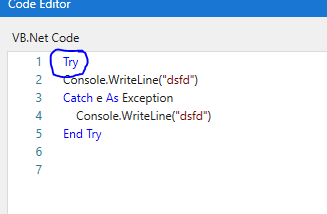# LOOPING FOR EACH IN INVOKE CODE

Try using the below code and see if it works.

For Each row As DataRow In Dt2.Rows
row(“Sign”) = CDbl(row(“amount”).tostring)*-1
row(“percent”) = CDbl(row(“sign”).tostring)*10
row(“total”) = CDbl(row(“percent”).tostring)-CDbl(row(“sign”).tostring)
Next

add this try catch block to get an exact information of the error

Try
For Each row As DataRow In Dt2.Rows
row(“Sign”) = CDbl(row(“amount”).tostring)*-1
row(“percent”) = CDbl(row(“sign”).tostring)*10
row(“total”) = CDbl(row(“percent”).tostring)-CDbl(row(“sign”).tostring)
Next
Catch e As Exception
console.WriteLine(e)

Regards.

The double quote ending in the 4th line after sign is not correct, delete that and add it again and check if it works.

Regards.

Could you share the screenshot of the code

Regards,
RPA Team.

Why are we using Invoke Code?
We can use direct for each row UiPath commands

directly i have done this
but i need to be done through invoke code with looping

Add Try at the First line like below. It shoudh fix that try errorCheck End Try is present at the end of the code

Follow the structure in my previous screenshot

Try
Catch e As Exception
End Try

try this code -

Try
For Each row As DataRow In Dt2.Rows
row(“Sign”) = CDbl(row(“amount”).tostring)*-1
row(“percent”) = CDbl(row(“sign”).tostring)*10
row(“total”) = CDbl(row(“percent”).tostring)-CDbl(row(“sign”).tostring)
Next
Catch e As Exception
console.WriteLine(e)
end try

could you share the code screenshot please.

Regards